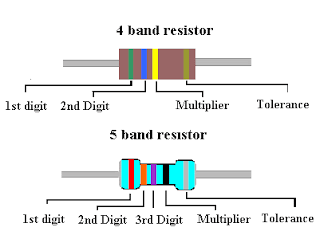## July 13, 2012

### Resistance Calculation From Color Code of a ResistorSymbol Of  Resistor
Now, we are going to discuss that how to find the resistance of a Resistor for its color bands. I performed this experiment in university lab.

The purpose of sharing this process is to help fellow students for preparation of their Physics lab manuals.

Keep reading this post for circuit diagrams and full range of all the value tables. This experiment was performed in the Campus Physics lab.

Experiment # 7

Resistance Calculation From Color Code & Verification Through Multi-Meter

Apparatus: Multi-meter, Battery, DC Power Supply, Jumper Wires, Breadboard, Color Code Chart, Function Generator.Resistance with Color Coding image courtesy: brassmein

Procedure:
1. Calculate the resistance of the available resistors by using color codes.
2. Switch the selector of multi-meter to resistance measurement.
3. Select the minimum range and measure resistance by connecting both probes of meter to the ends of resistors. Be sure, not to touch both ends of the resistor while the probes of meter are connected to the resistor. This will make a resistive path through your body in parallel with the resistor, thus affecting the correct reading. If the resistance of multi-meter pegged, i-e. Displays "1" on the left hand side of LCD display; this mean the resistance is greater than the selected range.
4. Switch to the next available range and measure the resistance again.
5. Repeat the procedure for different resistors available.
Resistor Color Code Chart:

Verification:

This is the simplest and easiest way to calculate the Resistance of a Resistor by its colors using color coding chart.